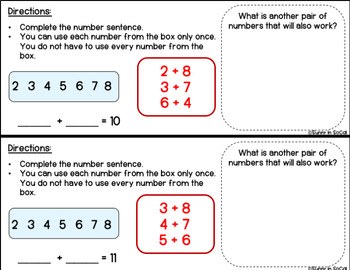# Math Challenges: Adding Within 20Subject
Resource Type
File Type

PDF

(1 MB|30 pages)
Product Rating
Standards
• Product Description
• StandardsNEW

This resource is a great way for students to build their flexibility with numbers while also engaging in critical thinking. Students will build these math skills through elimination computation. Each problem gives the sum, as well as a set of multiple numbers, and students work to figure which numbers can be added to create that sum.

The questions get progressively more difficult as the sums get larger, and include both 2 addends and 3 addends.

This makes a great math center, or an early finisher activity that keeps students engaged in critical math thinking.

Fluently add and subtract within 20 using mental strategies. By end of Grade 2, know from memory all sums of two one-digit numbers.
Determine the unknown whole number in an addition or subtraction equation relating three whole numbers. For example, determine the unknown number that makes the equation true in each of the equations 8 + ? = 11, 5 = ▯ - 3, 6 + 6 = ▯.
Add and subtract within 20, demonstrating fluency for addition and subtraction within 10. Use strategies such as counting on; making ten (e.g., 8 + 6 = 8 + 2 + 4 = 10 + 4 = 14); decomposing a number leading to a ten (e.g., 13 - 4 = 13 - 3 - 1 = 10 - 1 = 9); using the relationship between addition and subtraction (e.g., knowing that 8 + 4 = 12, one knows 12 - 8 = 4); and creating equivalent but easier or known sums (e.g., adding 6 + 7 by creating the known equivalent 6 + 6 + 1 = 12 + 1 = 13).
Total Pages
30 pages
Included
Teaching Duration
N/A
Report this Resource to TpT
Reported resources will be reviewed by our team. Report this resource to let us know if this resource violates TpT’s content guidelines.